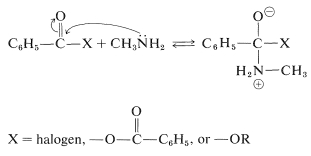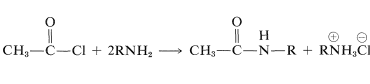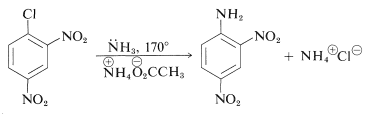# 23.9: Amines as Nucleophiles

$$\newcommand{\vecs}{\overset { \rightharpoonup} {\mathbf{#1}} }$$ $$\newcommand{\vecd}{\overset{-\!-\!\rightharpoonup}{\vphantom{a}\smash {#1}}}$$$$\newcommand{\id}{\mathrm{id}}$$ $$\newcommand{\Span}{\mathrm{span}}$$ $$\newcommand{\kernel}{\mathrm{null}\,}$$ $$\newcommand{\range}{\mathrm{range}\,}$$ $$\newcommand{\RealPart}{\mathrm{Re}}$$ $$\newcommand{\ImaginaryPart}{\mathrm{Im}}$$ $$\newcommand{\Argument}{\mathrm{Arg}}$$ $$\newcommand{\norm}{\| #1 \|}$$ $$\newcommand{\inner}{\langle #1, #2 \rangle}$$ $$\newcommand{\Span}{\mathrm{span}}$$ $$\newcommand{\id}{\mathrm{id}}$$ $$\newcommand{\Span}{\mathrm{span}}$$ $$\newcommand{\kernel}{\mathrm{null}\,}$$ $$\newcommand{\range}{\mathrm{range}\,}$$ $$\newcommand{\RealPart}{\mathrm{Re}}$$ $$\newcommand{\ImaginaryPart}{\mathrm{Im}}$$ $$\newcommand{\Argument}{\mathrm{Arg}}$$ $$\newcommand{\norm}{\| #1 \|}$$ $$\newcommand{\inner}{\langle #1, #2 \rangle}$$ $$\newcommand{\Span}{\mathrm{span}}$$$$\newcommand{\AA}{\unicode[.8,0]{x212B}}$$

## Acylation of Amines. Synthesis of Amides

The unshared electrons on nitrogen play a key role in the reactions of amines. In fact, almost all reactions of amines at the nitrogen atom have, as a first step, the formation of a bond involving the unshared electron pair on nitrogen. A typical example is acylation, which is amide formation through the reaction of an acyl chloride, an anhydride, or an ester with an amine. The initial step in these reactions with benzenecarbonyl derivatives and methanamine as illustrative reactants is as follows:The reaction is completed by loss of a proton and elimination of $$\ce{X}^\ominus$$:The reaction is called acylation because an acyl group, $$\ce{RCO}-$$, is transferred to the amine nitrogen. It will be seen that these reactions are very similar to the formation of esters by acylating agents, whereby the acyl group is transferred to the oxygen of an alcohol (Section 15-4D):A serious disadvantage to the preparation of amides through the reaction of an amine with an acyl chloride (or anhydride) is the formation of one mole of amine salt for each mole of amide:This is especially serious if the amine is the expensive ingredient in the reaction. In such circumstances, the reaction usually is carried on in a two-phase system wit the acyl chloride and amine in the nonaqueous phase and sodium hydroxide in the aqueous phase. As the amine salt is formed and dissolves in the water, it is converted back to amine by the sodium hydroxide and extracted back into the nonaqueous phase:

$\ce{R} \overset{\oplus}{\ce{N}} \ce{H_3} \overset{\ominus}{\ce{Cl}} + \overset{\oplus}{\ce{Na}} \overset{\ominus}{\ce{OH}} \rightarrow \ce{RNH_2} + \overset{\oplus}{\ce{Na}} \overset{\ominus}{\ce{Cl}} + \ce{H_2O}$

This procedure requires an excess of acid chloride because some of it is wasted by hydrolysis.

## Imine and Enamine Formation

Amines also add to the carbonyl carbon of aldehydes and ketones, but the reactions take a different course from acylation and, with ammonia or a primary amine, yield imines, $$\ce{-C=N-R}$$, as previously discussed in Section 16-4C.

Imines formed from ammonia and aldehydes $$\left( \ce{RCH=NH} \right)$$ are very unstable and readily polymerize (Section 16-4C). However, substitution of an alkyl or aryl group on the nitrogen increases the stability, and $$\ce{N}$$-substituted imines, $$\ce{-C=N-R}$$, are familiarly known as Schiff bases. They are key intermediates in a number of synthetic and biological reactions (see, for example, 17-3F) and are capable of rearrangement by reversible proton transfer that, in some respects, resembles the rearrangement of ketones to enols:Secondary amines cannot form imines with aldehydes and ketones but may react instead to form enamines, $$\ce{-C=C-NR_2}$$. The formation and synthetic uses of these compounds were discussed previously (Sections 16-4C, 17-4B, and 18-9D).

## Sulfonamide Formation from Amines

We have seen that amines react with acyl chlorides to give amides. A very similar reaction occurs with sulfonyl chlorides to give sulfonamides. An example is benzenesulfonyl chloride reacting with methanamine to give $$\ce{N}$$-methylbenzenesulfonamide:Sulfonylation of amines can be a useful way of differentiating (chemically) between primary, secondary, and tertiary amines by what is known as the Hinsberg test. Primary and secondary amines both react with a sulfonyl chloride, but only the sulfonamide from the primary amines has an $$\ce{N-H}$$ hydrogen. The sulfonyl group makes this hydrogen relatively acidic and the sulfonamide therefore dissolves readily in sodium hydroxide solutions. The secondary amine does not give a base-soluble amide, whereas the tertiary amine gives no sulfonamide:Sulfonamides have medicinal value as antibacterial agents. In fact, 4-aminobenzenesulfonamide was the first synthetic antibacterial drug in clinical use, and is effective against a large number of bacterial infections:This substance inhibits the growth of bacteria by interfering with the synthesis of folic acid, $$7$$, which is an essential substance for bacteria and animals alike. However, animals acquire folic acid from a normal diet, whereas bacteria have to synthesize it. Biosynthesis of folic acid is blocked by 4-aminobenzenesulfonamide, probably because of the structural similarity of the sulfonamide to 4-aminobenzoic acid, which is a normal ingredient in the biosynthesis of folic acid. The enzyme system involved apparently substitutes the sulfonamide for the aminobenzoic acid and creates a sulfonamide-type folic acid instead of the carboxamide derivative (compare structures $$7$$ and $$8$$):Some 10,000 structurally different sulfonamides have been synthesized as a result of the discovery of the antibacterial properties of sulfanilamide. The practice of synthesizing numerous structurally related compounds in an effort to find some that are more efficient or have fewer side effects than those already available is very important to the pharmaceutical industry. However, as is usually the case, of the many known sulfonamides only about thirty have the proper balance of qualities to be clinically useful.

## Alkylation. Synthesis of Alkanamides

Ammonia and amines can function as nucleophiles in $$S_\text{N}2$$ displacement reactions of alkyl halides (Section 8-7E). Such processes provide syntheses of alkanamines only with those halides that are reactive in $$S_\text{N}2$$ but not $$E2$$ reactions. For example,

$\ce{NH_3} + \ce{CH_3-I} \overset{S_\text{N}2}{\longrightarrow} \ce{CH_3-NH_3^+} \ce{I^-} \tag{23-5}$

The product formed according to Equation 23-5 is an ammonium salt from which the parent amine can be recovered by neutralization with a strong base, such as sodium hydroxide:

$\ce{CH_3NH_3^+} \ce{I^-} + \ce{Na^+} \ce{^-OH} \rightleftharpoons \ce{CH_3NH_2} + \ce{Na^+} \ce{I^-} + \ce{H_2O} \tag{23-6}$

Acid-base equilibria similar to Equation 23-6 also occur between an ammonium salt and a neutral amine (Equation 23-7). This can have serious consequences in amine alkylations because it can lead to mixtures of products, whereby more than one alkyl group is bonded to nitrogen:

\begin{align} \ce{CH_3NH_3^+} \ce{I^-} + \ce{NH_3} &\rightleftharpoons \ce{CH_3NH_2} + \ce{NH_4^+} \ce{I^-} \\ \ce{CH_3NH_2} + \ce{CH_3-I} &\rightarrow \ce{(CH_3)_2CH_2^+} \ce{I^-} \end{align} \tag{23-7}

Therefore we may expect the reaction of ammonia with methyl iodide to give four possible alkylation products, mono-, di-, and trimethylamines, as well as tetramethylammonium iodide:

$\ce{NH_3} \underset{-\ce{HI}}{\overset{\ce{CH_3I}}{\longrightarrow}} \ce{CH_3NH_2} \underset{-\ce{HI}}{\overset{\ce{CH_3I}}{\longrightarrow}} \ce{(CH_3)_2NH} \underset{-\ce{HI}}{\overset{\ce{CH_3I}}{\longrightarrow}} \ce{(CH_3)_3N} \overset{\ce{CH_3I}}{\longrightarrow} \ce{(CH_3)_4N}^\oplus \ce{I}^\ominus$

Despite the fact that alkylation reactions of amines generally give mixtures of products, they are of practical value on an industrial scale. The commercial synthesis of methanamines uses methanol as the methylating agent and aluminum oxide as an acidic catalyst; all three amines are formed, and are separated by distillation and extraction. The function of the catalyst is to make $$\ce{OH}$$ a better leaving group (Section 8-7D):

$\ce{CH_3OH} + \ce{NH_3} \underset{450^\text{o}, \: 200 \: \text{psi}}{\overset{\ce{Al_2O_3}}{\longrightarrow}} \ce{CH_3NH_2} + \ce{(CH_3)_2NH} + \ce{(CH_3)_3N} + \ce{H_2O}$

The tetraalkylammonium halides formed by complete alkylation of amines are ionic compounds that resemble alkali-metal salts. When silver oxide is used to precipitate the halide ion, tetraalkylammonium halides are converted to tetraalkylammonium hydroxides, which are strongly basic substances similar to sodium or potassium hydroxide:

$\ce{(CH_3)_4} \overset{\oplus}{\ce{N}} \: \overset{\ominus}{\ce{I}} \underset{-\ce{AgI}}{\overset{\ce{Ag_2O}, \: \ce{H_2O}}{\longrightarrow}} \ce{(CH_3)_4} \overset{\oplus}{\ce{N}} \: \overset{\ominus}{\ce{OH}}$

Higher-molecular-weight alkylammonium hydroxides decompose on heating to give alkenes. The reaction is a standard method for the preparation of alkenes and is known as the Hofmann elimination (see Section 8-8B):In principle, the same problems of polyalkylation and $$E2$$ elimination exist with the amine anion as with the neutral amine - and as far as $$E2$$ goes, much more so.

There are nitrogen anions that are useful in alkylation reactions, but they are derived from carboxamides and sulfonamides rather than amines. Two examples are given here to illustrate the synthesis of a primary and a secondary amine (also see Section 18-10C):

Gabriel synthesis of primary aminesThe success of the Gabriel synthesis depends on $$\ce{N}$$-alkylation being favored over $$\ce{O}$$-alkylation and $$S_\text{N}2$$ being favored over $$E2$$. Polar, aprotic solvents such as methylsufinylmethane, $$\ce{(CH_3)_2SO}$$, are useful for the Gabriel synthesis. Hydrolysis of the alkylation product often is difficult and "amide interchange" (analogous to ester interchange, Section 18-7A) with hydrazine can be an effective way to free the amine from the imide.

Sulfonamide synthesis of secondary aminesIn this synthesis, the acidic properties of sulfonamides of the type $$\ce{C_6H_5SO_2NHR}$$ are utilized to form anions capable of alkylation by the $$S_\text{N}2$$ mechanism.

## Arylation. Synthesis of Arenamines

In previous discussions (Section 14-6A) we stated that it is not possible to displace halogen from simple aryl halides such as bromobenzene by simple $$S_\text{N}2$$ reactions using amines or other weakly basic nucleophiles at ordinary temperatures:However, arylation with such systems will occur with strong bases by the benzyne mechanism (Sections 14-6C and 23-8).

Arylation of amines by the direct displacement of aryl halides is possible when the halogen is activated by strong electron-withdrawing groups in the ortho and para positions. For examples, 2,4-dinitrobenzenamine can be prepared by heating 2,4-dinitrochlorobenzene with ammonia:The reasons why this reaction proceeds are discussed in detail in Section 14-6B.

## Arenamines as Nucleophiles. Electrophilic Aromatic Substitution

The nitrogen of arenamines is less basic and less nucleophilic than the nitrogen of alkanamines because of electron delocalization of the nitrogen lone pair, as shown for benzenamine in Section 23-7C. The polar valence-bond structures emphasize that the ring atoms, particularly the ortho and para positions, should be more nucleophilic than in benzene. Accordingly, the amino group strongly activates the ring toward attack by electrophiles. In fact, bromine reacts rapidly with benzenamine in aqueous solution to introduce three bromine substituents and form 2,4,6-tribromobenzenamine; no catalyst is required:Weakly electrophilic reagents that do not normally attack benzene will attack the ring carbons of arenamines. Some of those reactions are described later in the chapter (Sections 23-10C and 23-10D).